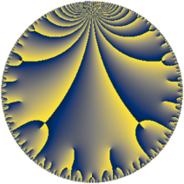Properties

 Label 126.9.qLevel $126$ Weight $9$ Character orbit 126.q Rep. character $\chi_{126}(29,\cdot)$ Character field $\Q(\zeta_{6})$ Dimension $96$ Newform subspaces $1$ Sturm bound $216$ Trace bound $0$

Related objects

Defining parameters

 Level: $$N$$ $$=$$ $$126 = 2 \cdot 3^{2} \cdot 7$$ Weight: $$k$$ $$=$$ $$9$$ Character orbit: $$[\chi]$$ $$=$$ 126.q (of order $$6$$ and degree $$2$$) Character conductor: $$\operatorname{cond}(\chi)$$ $$=$$ $$9$$ Character field: $$\Q(\zeta_{6})$$ Newform subspaces: $$1$$ Sturm bound: $$216$$ Trace bound: $$0$$

Dimensions

The following table gives the dimensions of various subspaces of $$M_{9}(126, [\chi])$$.

Total New Old
Modular forms 392 96 296
Cusp forms 376 96 280
Eisenstein series 16 0 16

Trace form

 $$96 q - 252 q^{3} + 6144 q^{4} + 1764 q^{5} - 1792 q^{6} + 34996 q^{9} + O(q^{10})$$ $$96 q - 252 q^{3} + 6144 q^{4} + 1764 q^{5} - 1792 q^{6} + 34996 q^{9} - 57348 q^{11} - 10752 q^{12} + 15076 q^{15} - 786432 q^{16} + 201216 q^{18} - 354144 q^{19} + 225792 q^{20} - 163268 q^{21} + 123648 q^{22} - 689760 q^{23} + 229376 q^{24} + 3073212 q^{25} - 361368 q^{27} + 3804948 q^{29} + 2023936 q^{30} - 1227660 q^{31} + 582764 q^{33} - 440832 q^{34} + 4911616 q^{36} - 8874600 q^{37} + 2628864 q^{38} + 3402136 q^{39} - 22740480 q^{41} - 2941680 q^{43} + 42836444 q^{45} + 56756700 q^{47} + 2752512 q^{48} - 39530064 q^{49} - 19413504 q^{50} - 67627672 q^{51} - 12194560 q^{54} - 17217816 q^{55} + 53464776 q^{57} - 91337652 q^{59} - 1799168 q^{60} + 20571768 q^{63} - 201326592 q^{64} + 57140244 q^{65} + 26733056 q^{66} - 15593508 q^{67} - 5289984 q^{68} - 69818224 q^{69} + 28114944 q^{72} - 32396448 q^{73} - 51351552 q^{74} - 132131048 q^{75} - 22665216 q^{76} + 23031296 q^{78} - 22922628 q^{79} + 78498364 q^{81} + 274692096 q^{82} - 228400452 q^{83} - 41796608 q^{84} + 194191044 q^{85} + 270220032 q^{86} - 134502200 q^{87} - 15826944 q^{88} - 289354240 q^{90} + 25181688 q^{91} - 88289280 q^{92} - 437969660 q^{93} + 354685536 q^{95} + 58720256 q^{96} + 293960100 q^{97} - 1181735648 q^{99} + O(q^{100})$$

Decomposition of $$S_{9}^{\mathrm{new}}(126, [\chi])$$ into newform subspaces

Label Dim. $$A$$ Field CM Traces $q$-expansion
$a_{2}$ $a_{3}$ $a_{5}$ $a_{7}$
126.9.q.a $96$ $51.330$ None $$0$$ $$-252$$ $$1764$$ $$0$$

Decomposition of $$S_{9}^{\mathrm{old}}(126, [\chi])$$ into lower level spaces

$$S_{9}^{\mathrm{old}}(126, [\chi]) \cong$$ $$S_{9}^{\mathrm{new}}(9, [\chi])$$$$^{\oplus 4}$$$$\oplus$$$$S_{9}^{\mathrm{new}}(18, [\chi])$$$$^{\oplus 2}$$$$\oplus$$$$S_{9}^{\mathrm{new}}(63, [\chi])$$$$^{\oplus 2}$$